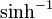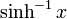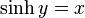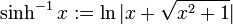# Inverse hyperbolic sine function

The inverse hyperbolic sine function, denoted$\sinh^{-1}$, is defined in the following equivalent ways:
1. It is the inverse function to the hyperbolic sine function, i.e.,$\sinh^{-1}x$ is defined as the unique real number$y$ such that$\sinh y = x$.$\sinh^{-1} x := \ln|x + \sqrt{x^2 + 1}|$